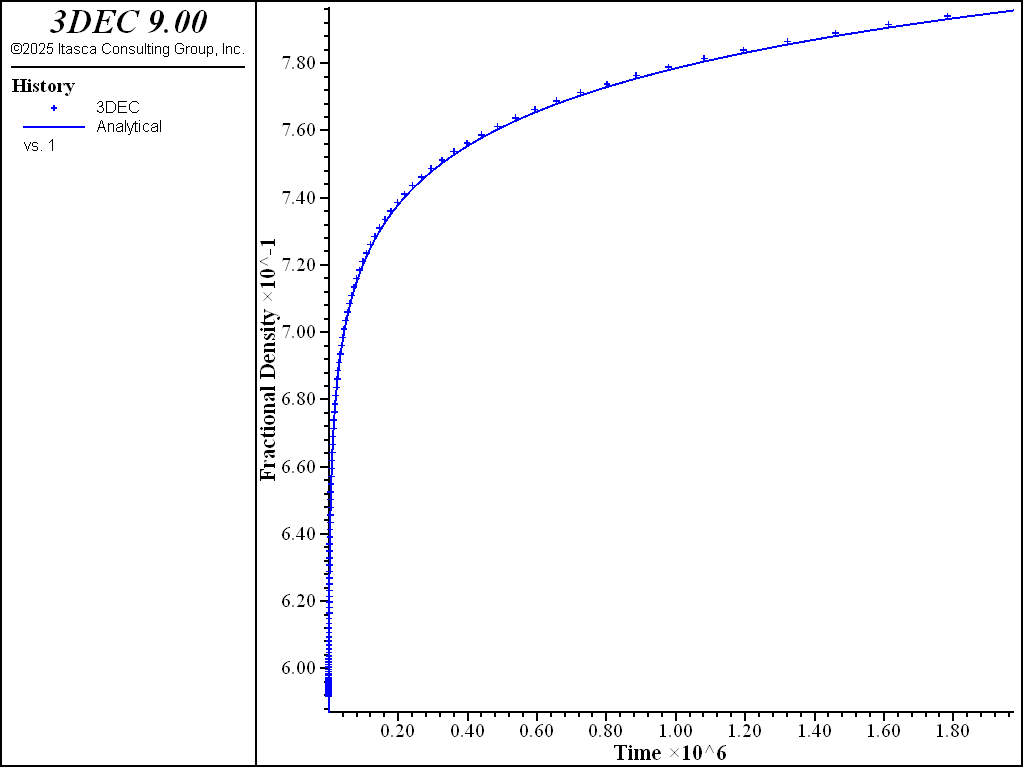# WIPP-Salt Model: Shear Compression Test

Note

To view this project in 3DEC, use the menu command Help ► Examples…. Choose “CreepMaterialModels/ ShearCrushedSalt” and select “ShearCrushedSalt.prj” to load. The project’s main data files are shown at the end of this example.

Results of the shear compression test here are presented to validate the WIPP-Salt model in 3DEC. The test involves one zone at an initially elastic state of equilibrium. The zone is allowed to creep for a period of 23 days, during which time the fractional density is monitored and compared to the analytical calculation.

In the shear compression test, both axial and confining stresses are kept constant. Using a notation convention in which $$\sigma_1$$ refers to the most negative (major) compressive stress and $$\sigma_3$$ refers to the least negative (minor) compressive stress, which is also the confining stress, then the stress invariants are

(1)$\sigma = {{1}\over{3}} (\sigma_1 + 2 \sigma_3)$

and

(2)$\bar\sigma = \sigma_3 - \sigma_1$

Also, we have

(3)$\sigma_{zz}^d = - {{2}\over{3}} (\sigma_3 - \sigma_1)$

and

(4)$\sigma_{xx}^d = \sigma_{yy}^d = {{1}\over{3}} (\sigma_3 - \sigma_1)$

For constant applied stresses, neglecting the creep component, the total strain rate equals the compaction strain rate, and we may write, using $$\beta$$ = 1 in the Equation on $$\dot\epsilon_{ij}^c$$ in WIPP-Salt model,

(5)$\dot\epsilon_{ij} = \dot\epsilon_v^c \left[{{\delta_{ij}}\over{3}} - {{\sigma_{ij}^d}\over{\bar{\sigma}}} \right]$

where $$\dot\epsilon_v^c$$ is given in WIPP-Salt model.

Substitution of Equation (1) to Equation (4) in Equation (5) yields

(6)$\dot{\epsilon_{zz}} = \dot{\epsilon_v^d}$
(7)$\dot{\epsilon_{xx}} = \dot{\epsilon_{yy}} = 0$

and no lateral compaction is predicted in this test for the constraints of the model.

The analytical expression for the fractional density is similar to that obtained for the hydrostatic compression test.

Two tests are performed: an unconfined compression test and a biaxial compression test. In both tests, $$\sigma_1$$ = -8.97 MPa. $$\sigma_3$$ = 0.0 in the unconfined compression test, and $$\sigma_3$$ = -6.90 MPa in the biaxial compression test. Initial and final density, and shear and bulk moduli are identical to those adopted in the hydrostatic compression test.

Numerical values of fractional density are compared to analytical values versus creep time for the unconfined compression test in Figure 1, and for the biaxial compression test in Figure 2. The error in fractional density is less than 0.1%. The histories of axial and lateral displacements for the unconfined compression test are shown in Figure 3, and for the biaxial compression test in Figure 4. As expected, no lateral displacement is calculated in these tests.Figure 1: Comparison of numerical and analytical prediction for fractional density for uniaxial compression.Figure 2: Comparison of numerical and analytical prediction for fractional density for biaxial compression.Figure 3: Histories of axial and lateral displacement for uniaxial compression.Figure 4: Histories of axial and lateral displacement for biaxial compression.

Data Files

ShearCrushedSalt.dat

model new
; File: ShearCrushedSalt.dat
; Shear compression of crushed salt
; --- constants ---
fish define cons
global valsig3 = 0.0 ; unconfined compression
global valsig1 = -8.97e6
global valsig = (valsig1 + 2.0 * valsig3) / 3.0
global valb0  = 1.3e8
global valb1  = 0.82e-6
global valb2  = -1.72e-2
global valrho = 1350.
global valrhof= 2300.
global valbf  = 58.6383e9
global valsf  = 35.3163e9
global valterm = valb0 * (math.exp(-valb1*valsig)-1.)
global valcons = math.exp(-valb2*valrho)
end
[cons]
;
; --- geometry ---
model configure creep
model large-strain off

block create brick 0 5 0 5 0 5
block zone gen edge 2.5
block zone cmodel assign wipp-salt
block zone property bulk 1.186E8 shear 7.14E7 young 1.784E8 poisson 0.2493 activation-energy 1.2E4 ...
constant-a 4.56 constant-b 127 constant-d 5.79E-36 creep-rate-critical 5.39E-8 constant-gas 1.987 ...
exponent 4.9 temperature 300 density [valrho] bulk-final [valbf] shear-final [valsf] density-final [valrhof] ...
compaction-0 [valb0] compaction-1 [valb1] compaction-2 [valb2] density-salt [valrho]
;
program call 'solution.fis'

; --- histories ---
history interval 100
model history creep time-total
model history timestep
fish history c_frd
fish history c_frdsol
fish history c_err
fish history c_ev
fish history c_evsol
fish history c_rbulk
block history displacement-x position 5 0 0
block history displacement-x position 5 0 5
block history displacement-z position 0 0 5
block history displacement-z position 5 0 5
;
model creep timestep starting 5.e-7
model creep timestep minimum 5.e-7
model creep timestep maximum 5e4
model creep timestep lower-multiplier 1.001
model creep timestep automatic

model save 'crushed_salt_ini'

; --------- Uniaxial -------------------
;
block face apply stress [valsig3] 0.0 0.0 0 0 0 range position-x 5
block face apply stress 0.0 [valsig3] 0.0 0 0 0 range position-y 5
block face apply stress 0.0 0.0 [valsig1] 0 0 0 range position-z 5
block gridpoint apply velocity-x 0 range position-x 0
block gridpoint apply velocity-y 0 range position-y 0
block gridpoint apply velocity-z 0 range position-z 0

; --- initial conditions ---
block insitu stress [valsig3] [valsig3] [valsig1] 0 0 0

model solve time-total 2e6
;
model save 'crushed_salt-uniaxial'
;
; --------- Biaxial ------------------------
model restore 'crushed_salt_ini'

[valsig3 = -6.90e6]
[cons]

block face apply stress [valsig3] 0.0 0.0 0 0 0 range position-x 5
block face apply stress 0.0 [valsig3] 0.0 0 0 0 range position-y 5
block face apply stress 0.0 0.0 [valsig1] 0 0 0 range position-z 5
block gridpoint apply velocity-x 0 range position-x 0
block gridpoint apply velocity-y 0 range position-y 0
block gridpoint apply velocity-z 0 range position-z 0

; --- initial conditions ---
block insitu stress [valsig3] [valsig3] [valsig1] 0 0 0

model solve time-total 2e6
;
model save 'crushed_salt-biaxial'